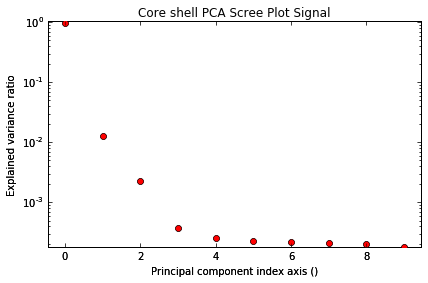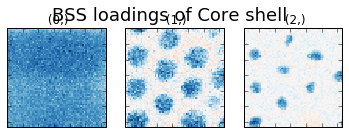# Online Robust PCA¶

### Tom Furnival ([email protected])¶

14th July 2016

A key assumption of using Principal Component Analysis (PCA) for denoising and dimensionality reduction is that the underlying data is corrupted by a small amount of Gaussian noise. As a result, PCA is very sensitive to large errors, such as X-ray spikes, dead pixels or even missing data.

Robust PCA (RPCA) was introduced to overcome this problem . It decomposes a corrupted dataset Y into a low-rank component X and a sparse error component E, i.e. Y = X + E.

This notebook is a demonstration of the Online Robust PCA (ORPCA) algorithm , as implemented in HyperSpy, which is able to recover the low-rank component of a corrupted matrix in a memory-efficient manner that is suitable for "big data". In particular, the algorithm updates the low-rank estimate as each observation arrives, avoiding the expensive re-calculation associated with batch methods.

 E. J. Candes et al, Robust Principal Component Analysis?, (2009).
URL: https://statweb.stanford.edu/~candes/papers/RobustPCA.pdf

 J. Feng, H. Xu and S. Yan, "Online Robust PCA via Stochastic Optimization", (2013).
URL: https://papers.nips.cc/paper/5131-online-robust-pca-via-stochastic-optimization

In :
#Download the data (1MB)
from urllib.request import urlretrieve, urlopen
from zipfile import ZipFile
files = urlretrieve("https://www.dropbox.com/s/ecdlgwxjq04m5mx/HyperSpy_demos_EDS_TEM_files.zip?raw=1", "./HyperSpy_demos_EDX_TEM_files.zip")
with ZipFile("HyperSpy_demos_EDX_TEM_files.zip") as z:
z.extractall()

# Set up HyperSpy
import hyperspy.api as hs
import numpy as np
import matplotlib.pyplot as plt

# Let's go with inline plots
%matplotlib inline


## 1. Normal PCA+ICA¶

In the first instance, we follow the routine described in this HyperSpy tutorial, which carries out the standard PCA decomposition to reduce noise, followed by BSS with a known number of components (3).

In :
# Load data
cs.change_dtype('float')
cs.plot()

# Apply PCA
cs.decomposition()
cs.plot_explained_variance_ratio()

# Apply ICA
cs.blind_source_separation(3)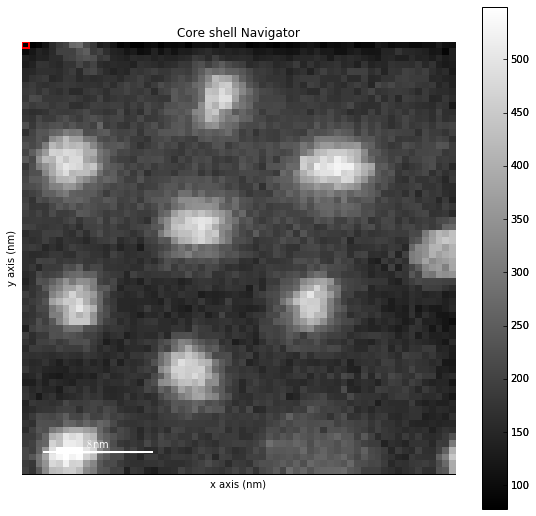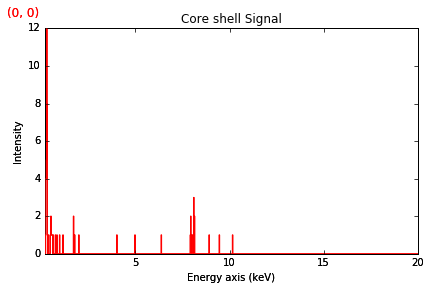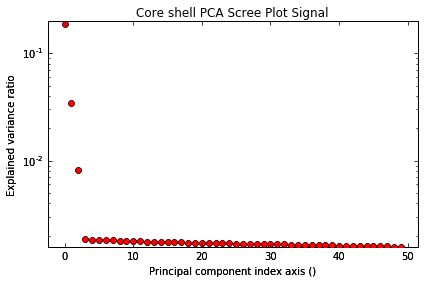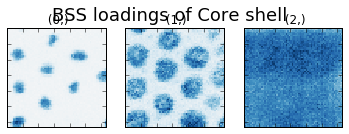## 2. Normal PCA+ICA on corrupted data¶

Now we corrupt the data with sparse errors to simulate e.g. hot pixels or X-ray spikes. We apply the corruption by masking 5% of the datapoints with the value 1000.

In :
# Corrupt the data with sparse errors
sparse = 0.05 # Fraction of corrupted data

cserror = cs.copy()
E = 1000 * np.random.binomial(1, sparse, cs.data.shape)
cserror.data = cserror.data + E

cserror.plot()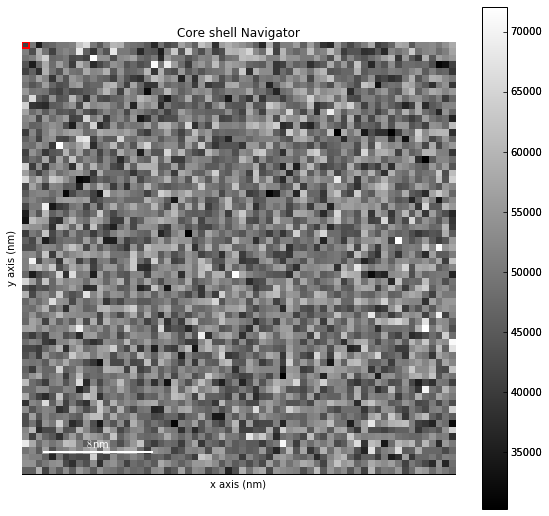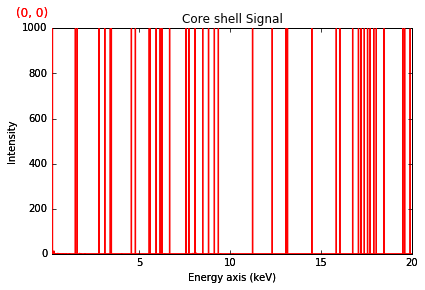As discussed above, PCA is known to be sensitive to outliers, so first we try the same PCA+ICA analysis. Notice how the scree plot is dominated by the sparse errors and so is tricky to interpret. The BSS results are essentially noise.

In :
# Apply PCA
cserror.decomposition()
cserror.plot_explained_variance_ratio()

# Apply ICA
cserror.blind_source_separation(3)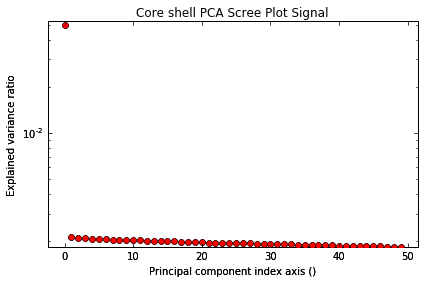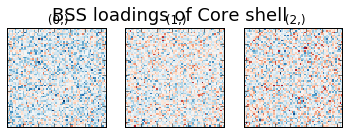## 3. Online Robust PCA+ICA on corrupted data¶

Now we try the ORPCA algorithm on the same corrupted data, followed by ICA.

• output_dimension is an initial estimate of the rank of the data
• init is the initialization method, which can be
- rand (random), or
- qr (QR-decomposition based) - default
• lambda1 is the nuclear norm regularization parameter
• lambda2 is the sparse error regularization parameter

Here we set normalize_poissonian_noise=False, although this option may depend on your data.

Interpreting the scree plot is much more straightforward, and the results of the BSS match the originals seen above.

In :
# Try online robust PCA
cserror.decomposition(normalize_poissonian_noise=False,
algorithm='ORPCA',
output_dimension=10,
init='rand',
lambda1=0.005,
lambda2=0.005)
cserror.plot_explained_variance_ratio()

# Apply ICA
cserror.blind_source_separation(3)